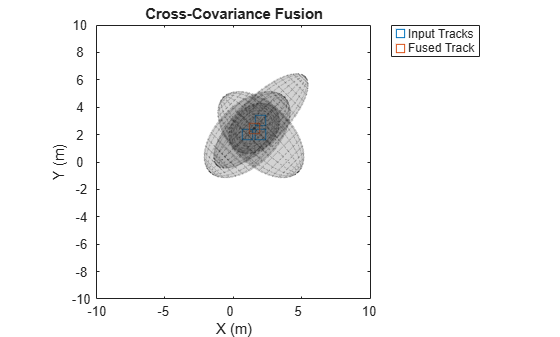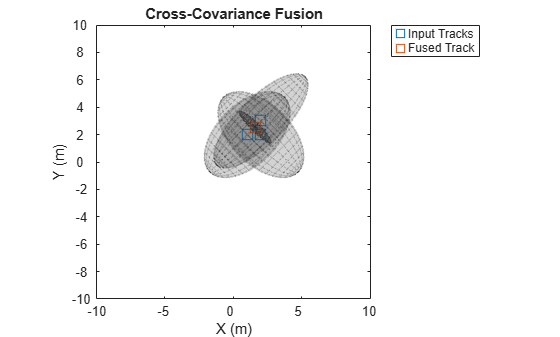Documentation

fusexcov

Covariance fusion using cross-covariance

Description

example

[fusedState,fusedCov] = fusexcov(trackState,trackCov) fuses the track states in trackState and their corresponding covariance matrices trackCov. The function estimates the fused state and covariance within a Bayesian framework in which the cross-correlation between tracks is unknown.

example

[fusedState,fusedCov] = fusexcov(trackState,trackCov,crossCovFactor) specifies a cross-covariance factor for the effective correlation coefficient when computing the cross-covariance.

Examples

collapse all

Define a state vector of tracks.

x(:,1) = [1;2;0];
x(:,2) = [2;2;0];
x(:,3) = [2;3;0];

Define the covariance matrices of the tracks.

p(:,:,1) = [10 5 0; 5 10 0;0 0 1];
p(:,:,2) = [10 -5 0; -5 10 0;0 0 1];
p(:,:,3) = [12 9 0; 9 12 0;0 0 1];

Estimate the fused state vector and its covariance.

[fusedState,fusedCov] = fusexcov(x,p);

Use trackPlotter to plot the results.

tPlotter = theaterPlot('XLim',[-10 10],'YLim',[-10 10],'ZLim',[-10 10]);
tPlotter1 = trackPlotter(tPlotter, ...
'DisplayName','Input Tracks','MarkerEdgeColor',[0.000 0.447 0.741]);
tPlotter2 = trackPlotter(tPlotter, ...
'DisplayName','Fused Track','MarkerEdgeColor',[0.850 0.325 0.098]);
plotTrack(tPlotter1,x',p)
plotTrack(tPlotter2, fusedState', fusedCov)
title('Cross-Covariance Fusion')Define a state vector of tracks.

x(:,1) = [1;2;0];
x(:,2) = [2;2;0];
x(:,3) = [2;3;0];

Define the covariance matrices of the tracks.

p(:,:,1) = [10 5 0; 5 10 0;0 0 1];
p(:,:,2) = [10 -5 0; -5 10 0;0 0 1];
p(:,:,3) = [12 9 0; 9 12 0;0 0 1];

Estimate the fused state vector and its covariance. Specify a cross-covariance factor of 0.5.

[fusedState,fusedCov] = fusexcov(x,p,0.5);

Use trackPlotter to plot the results.

tPlotter = theaterPlot('XLim',[-10 10],'YLim',[-10 10],'ZLim',[-10 10]);
tPlotter1 = trackPlotter(tPlotter, ...
'DisplayName','Input Tracks','MarkerEdgeColor',[0.000 0.447 0.741]);
tPlotter2 = trackPlotter(tPlotter, ...
'DisplayName','Fused Track','MarkerEdgeColor',[0.850 0.325 0.098]);
plotTrack(tPlotter1,x',p)
plotTrack(tPlotter2, fusedState', fusedCov)
title('Cross-Covariance Fusion')Input Arguments

collapse all

Track states, specified as an N-by-M matrix, where N is the dimension of the state and M is the number of tracks.

Data Types: single | double

Track covariance matrices, specified as an N-by-N-by-M array, where N is the dimension of the state and M is the number of tracks.

Data Types: single | double

Cross-covariance factor, specified as a scalar.

Data Types: single | double

Output Arguments

collapse all

Fused state, returned as an N-by-1 vector, where N is the dimension of the state.

Fused covariance matrix, returned as an N-by-N matrix, where N is the dimension of the state.

Extended Capabilities

C/C++ Code GenerationGenerate C and C++ code using MATLAB® Coder™.